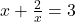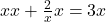##solve for x must be 2 variables that equal to x Please put the steps.

Questionsolve for x
must be 2 variables that equal to x

in progress 0
4 weeks 2021-08-18T03:42:34+00:00 1 Answers 0 views 0

x=2, x=1

Step-by-step explanation:

Multiply both sides by x, so you get (x^2)+2=3x, which comes fromSolve for (x^2)+2=3x

(x^2)-3x+2=0

(x-2)(x-1)=0, use box method or FOIL if needed

x=2,x=1